# 九种查找算法C语言与CPP编程

﻿

## 时间、空间复杂度比较

﻿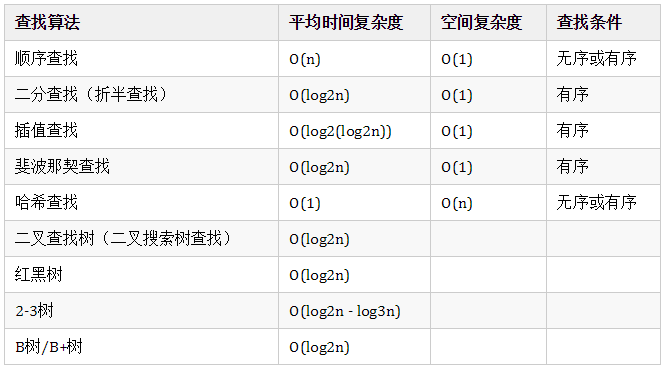## 1 顺序查找

#include <stdio.h>#include <stdlib.h>#define keyType int//2020.05.24typedef struct{	keyType key;//查找表中每个数据元素的值}ElemType;typedef struct{	ElemType *elem;//存放查找表中数据元素的数组	int length;//记录查找表中数据的总数量}SSTable;//创建查询数据void Create(SSTable **st,int length){	(*st)=(SSTable*)malloc(sizeof(SSTable));	(*st)->length=length;	(*st)->elem =(ElemType*)malloc((length+1)*sizeof(ElemType));	printf("输入表中的数据元素：\n");	//根据查找表中数据元素的总长度，在存储时，从数组下标为 1 的空间开始存储数据	for (int i=1; i<=length; i++)	{		scanf("%d",&((*st)->elem[i].key));	}}//顺序查找函数，其中key为要查找的元素int Search_seq(SSTable *str,keyType key){	//str->elem.key=key;//将关键字作为一个数据元素存放到查找表的第一个位置，起监视哨的作用	int len = str->length;	//从最后一个数据元素依次遍历，一直遍历到数组下标为0	for(int i=1; i<len+1; i++)   //创建数据从数组下标为1开始，查询也从1开始	{		if(str->elem[i].key == key)		{			return i;		}	}	//如果 i=0，说明查找失败；查找成功返回要查找元素key的位置i	return 0;}int main(){	SSTable *str;	int num;	printf("请输入创建数据元素的个数：\n");	scanf("%d",&num);	Create(&str, num);	getchar();	printf("请输入要查找的数据：\n");	int key;	scanf("%d",&key);	int location=Search_seq(str, key);	if (location==0) {		printf("查找失败");	}else{		printf("要查找的%d的顺序为：%d",key,location);	}	return 0;}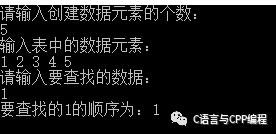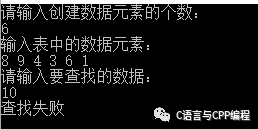## 2 二分查找（折半查找）

1. 确定查找范围low=0，high=N-1，计算中项mid=（low+high）/2。

2. 若mid==x或low>=high,则结束查找；否则，向下继续。

3. 若amid<x,说明待查找的元素值只可能在比中项元素大的范围内，则把mid+1的值赋给low，并重新计算mid，转去执行步骤2；若mid>x，说明待查找的元素值只可能在比中项元素小的范围内，则把mid-1的值赋给higt，并重新计算mid，转去执行步骤2。

• 查找元素必须是有序的，如果是无序的则要先进行排序操作。

• 在做查找的过程中，如果 low 指针和 high 指针的中间位置在计算时位于两个关键字中间，即求得 mid 的位置不是整数，需要统一做取整操作。

#include <stdio.h>#include <stdlib.h>#define keyType inttypedef struct{	keyType key;//查找表中每个数据元素的值}ElemType;typedef struct{	ElemType *elem;//存放查找表中数据元素的数组	int length;//记录查找表中数据的总数量}SSTable;//创建查询数据void Create(SSTable **st,int length){	(*st)=(SSTable*)malloc(sizeof(SSTable));	(*st)->length=length;	(*st)->elem =(ElemType*)malloc((length+1)*sizeof(ElemType));	printf("输入表中的数据元素：\n");	//根据查找表中数据元素的总长度，在存储时，从数组下标为 1 的空间开始存储数据	for (int i=1; i<=length; i++)	{		scanf("%d",&((*st)->elem[i].key));	}}//折半查找函数 key为要查找的元素int Search_Bin(SSTable *str,keyType key){	int low=1;//初始状态 low 指针指向第一个关键字	int high=str->length;//high 指向最后一个关键字	int mid;	while (low<=high)	{		mid=(low+high)/2;//int 本身为整形，所以，mid 每次为取整的整数		if(str->elem[mid].key==key)//如果 mid 指向的同要查找的相等，返回 mid 所指向的位置		{			return mid;		}        else if(str->elem[mid].key>key)//如果mid指向的关键字较大，则更新 high 指针的位置		{			high=mid-1;		}		//反之，则更新 low 指针的位置		else		{			low=mid+1;		}	}	return 0;}int main(){	SSTable *str;	int num;	printf("请输入创建数据元素的个数：\n");	scanf("%d",&num);	Create(&str, num);	getchar();	printf("请输入要查找的数据：\n");	int key;	scanf("%d",&key);	int location=Search_Bin(str, key);	if (location==0) {		printf("没有查找到");	}else{		printf("要查找的%d的顺序为：%d",key,location);	}	return 0;}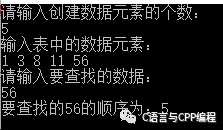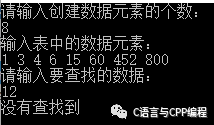## 3 插值查找

1. 确定查找范围low=0，high=N-1，计算中项mid=low+(key-a[low])/(a[high]-a[low])*(high-low)。

2. 若mid==x或low>=high,则结束查找；否则，向下继续。

3. 若amid<x,说明待查找的元素值只可能在比中项元素大的范围内，则把mid+1的值赋给low，并重新计算mid，转去执行步骤2；若mid>x，说明待查找的元素值只可能在比中项元素小的范围内，则把mid-1的值赋给higt，并重新计算mid，转去执行步骤2。

• 插值查找是基于折半查找进行了优化的查找方法。

• 当表长较大，而关键字分布又比较均匀的查找表来说，插值查找算法的平均性能要比折半查找要好得多。

#include<stdio.h>int array = { 1, 4, 9, 16, 27, 31, 33, 35, 45, 64 };int InsertionSearch(int data){    int low = 0;    int high = 10 - 1;    int mid = -1;    int comparisons = 1;    int index = -1;        while(low <= high)    {       printf("比较 %d 次\n" , comparisons );       printf("low : %d, list[%d] = %d\n", low, low, array[low]);       printf("high : %d, list[%d] = %d\n", high, high, array[high]);       comparisons++;       mid = low + (((double)(high - low) / (array[high] - array[low])) * (data - array[low]));       printf("mid = %d\n",mid);              // 没有找到       if(array[mid] == data)       {	   index = mid;           break;        }	else	{	   if(array[mid] < data)           {               low = mid + 1;           }           else	   {	       high = mid - 1;	    }	 }     }     printf("比较次数: %d\n", --comparisons);     return index;}int main(){    int location = InsertionSearch(27);  //测试代，查27，可以找到    if(location != -1)    {	printf("查找元素顺序为: %d\n" ,(location+1));     }     else     {         printf("没有查找到");     }     return 0;}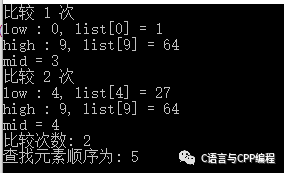## 4 斐波那契查找

1. 相等，mid位置的元素即为所求

2. 大于，low=mid+1,k-=2;

3. 小于，high=mid-1,k-=1。

low=mid+1说明待查找的元素在[mid+1,high]范围内，k-=2 说明范围[mid+1,high]内的元素个数为n-(F(k-1))= Fk-1-F(k-1)=Fk-F(k-1)-1=F(k-2)-1个，所以可以递归的应用斐波那契查找。

#include "stdafx.h"#include <memory>#include  <iostream>using namespace std;const int max_size=20;//斐波那契数组的长度/*构造一个斐波那契数组*/void Fibonacci(int * F){    F=0;    F=1;    for(int i=2;i<max_size;++i)        F[i]=F[i-1]+F[i-2];}/*定义斐波那契查找法*/int FibonacciSearch(int *a, int n, int key)  //a为要查找的数组,n为要查找的数组长度,key为要查找的关键字{  int low=0;  int high=n-1;    int F[max_size];  Fibonacci(F);//构造一个斐波那契数组F  int k=0;  while(n>F[k]-1)//计算n位于斐波那契数列的位置      ++k;  int  * temp;//将数组a扩展到F[k]-1的长度  temp=new int [F[k]-1];  memcpy(temp,a,n*sizeof(int));  for(int i=n;i<F[k]-1;++i)     temp[i]=a[n-1];    while(low<=high)  {    int mid=low+F[k-1]-1;    if(key<temp[mid])    {      high=mid-1;      k-=1;    }    else if(key>temp[mid])    {     low=mid+1;     k-=2;    }    else    {       if(mid<n)           return mid; //若相等则说明mid即为查找到的位置       else           return n-1; //若mid>=n则说明是扩展的数值,返回n-1    }  }  delete [] temp;  return 0;}int main(){    int a[] = {0,1,4,35,47,53,62,78,88,99};    int key=47;    int index=FibonacciSearch(a,sizeof(a)/sizeof(int),key);	if(index == 0)	{	   cout<<"没有找到"<<key;	}	else	{       cout<<key<<" 的位置是:"<<index;	}    return 0;}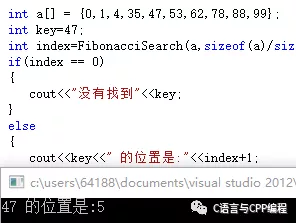47的位置为5

## 5 哈希查找

"直接定址"与"解决冲突"是哈希表的两大特点。

1. 用给定的哈希函数构造哈希表；

2. 根据选择的冲突处理方法(常见方法：拉链法和线性探测法)解决地址冲突；

3. 在哈希表的基础上执行哈希查找；

#include<stdio.h>#include<stdlib.h>#define SUCCESS 1#define UNSUCCESS 0#define OVERFLOW -1#define OK 1#define ERROR -1#define MAXNUM 9999		// 用于初始化哈希表的记录 keytypedef int Status;typedef int KeyType;// 哈希表中的记录类型typedef struct{	KeyType key;}RcdType;// 哈希表类型typedef struct{	RcdType *rcd;	int size;	int count;	int *tag;}HashTable;// 哈希表每次重建增长后的大小int hashsize[] = { 11, 31, 61, 127, 251, 503 };int index = 0;// 初始哈希表Status InitHashTable(HashTable &H, int size){	int i;	H.rcd = (RcdType *)malloc(sizeof(RcdType)*size);	H.tag = (int *)malloc(sizeof(int)*size);	if (NULL == H.rcd || NULL == H.tag) return OVERFLOW;	KeyType maxNum = MAXNUM;	for (i = 0; i < size; i++)	{		H.tag[i] = 0;		H.rcd[i].key = maxNum;	}	H.size = size;	H.count = 0;	return OK;}// 哈希函数：除留余数法int Hash(KeyType key, int m){	return (3 * key) % m;}// 处理哈希冲突：线性探测void collision(int &p, int m){	p = (p + 1) % m;}// 在哈希表中查询Status SearchHash(HashTable H, KeyType key, int &p, int &c){	p = Hash(key, H.size);	int h = p;	c = 0;	while ((1 == H.tag[p] && H.rcd[p].key != key) || -1 == H.tag[p])	{		collision(p, H.size);  c++;	}	if (1 == H.tag[p] && key == H.rcd[p].key) return SUCCESS;	else return UNSUCCESS;}//打印哈希表void printHash(HashTable H){	int  i;	printf("key : ");	for (i = 0; i < H.size; i++)		printf("%3d ", H.rcd[i].key);	printf("\n");	printf("tag : ");	for (i = 0; i < H.size; i++)		printf("%3d ", H.tag[i]);	printf("\n\n");}// 函数声明：插入哈希表Status InsertHash(HashTable &H, KeyType key);// 重建哈希表Status recreateHash(HashTable &H){	RcdType *orcd;	int *otag, osize, i;	orcd = H.rcd;	otag = H.tag;	osize = H.size;	InitHashTable(H, hashsize[index++]);	//把所有元素，按照新哈希函数放到新表中	for (i = 0; i < osize; i++)	{		if (1 == otag[i])		{			InsertHash(H, orcd[i].key);		}	}	return OK;}// 插入哈希表Status InsertHash(HashTable &H, KeyType key){	int p, c;	if (UNSUCCESS == SearchHash(H, key, p, c))	{ //没有相同key		if (c*1.0 / H.size < 0.5)		{ //冲突次数未达到上线			//插入代码			H.rcd[p].key = key;			H.tag[p] = 1;			H.count++;			return SUCCESS;		}		else recreateHash(H); //重构哈希表	}	return UNSUCCESS;}// 删除哈希表Status DeleteHash(HashTable &H, KeyType key){	int p, c;	if (SUCCESS == SearchHash(H, key, p, c))	{		//删除代码		H.tag[p] = -1;		H.count--;		return SUCCESS;	}	else return UNSUCCESS;}int main(){	printf("-----哈希表-----\n");	HashTable H;	int i;	int size = 11;	KeyType array = { 22, 41, 53, 46, 30, 13, 12, 67 };	KeyType key;	//初始化哈希表	printf("初始化哈希表\n");	if (SUCCESS == InitHashTable(H, hashsize[index++])) printf("初始化成功\n");	//插入哈希表	printf("插入哈希表\n");	for (i = 0; i <= 7; i++)	{		key = array[i];		InsertHash(H, key);		printHash(H);	}	//删除哈希表	printf("删除哈希表中key为12的元素\n");	int p, c;	if (SUCCESS == DeleteHash(H, 12))	{		printf("删除成功，此时哈希表为：\n");		printHash(H);	}	//查询哈希表	printf("查询哈希表中key为67的元素\n");	if (SUCCESS == SearchHash(H, 67, p, c)) printf("查询成功\n");	//再次插入，测试哈希表的重建	printf("再次插入，测试哈希表的重建：\n");	KeyType array1 = { 27, 47, 57, 47, 37, 17, 93, 67 };	for (i = 0; i <= 7; i++)	{		key = array1[i];		InsertHash(H, key);		printHash(H);	}	getchar();	return 0;}

## 6 二叉树查找

1. 若b是空树，则搜索失败：

2. 若x等于b的根节点的数据域之值，则查找成功：

3. 若x小于b的根节点的数据域之值，则搜索左子树：

4. 查找右子树。

//非递归查找算法BSTNode *BST_Search(BiTree T,ElemType key,BSTNode *&p){    //查找函数返回指向关键字值为key的结点指针，不存在则返回NULL    p=NULL;    while(T!=NULL&&key!=T->data)    {        p=T;                          //指向被查找结点的双亲        if(key<T->data)            T=T->lchild;              //查找左子树        else            T=T->rchild;              //查找右子树    }    return T;}//递归算法Status Search_BST(BiTree T, int key, BiTree f, BiTree *p){    //查找BST中是否存在key，f指向T双亲，其初始值为NULL    //若查找成功，指针p指向数据元素结点，返回true；    //若失败，p指向查找路径上访问的最后一个结点并返回false    if(!T)    {        *p=f;        return false;    }    else if(key==T->data)    {                      //查找成功        *p=T;        return true;    }    else if(key<T->data)        return Search_BST(T->lchild,key,T,p);   //递归查找左子树    else        return Search_BST(T->rchild,key,T,p);   //递归查找右子树    }

## 7 2-3树

2-3树运行每个节点保存1个或者两个的值。对于普通的2节点(2-node)，他保存1个key和左右两个自己点。对应3节点(3-node)，保存两个Key，2-3查找树的定义如下：

1. 要么为空，要么：

2. 对于2节点，该节点保存一个key及对应value，以及两个指向左右节点的节点，左节点也是一个2-3节点，所有的值都比key要小，右节点也是一个2-3节点，所有的值比key要大。

3. 对于3节点，该节点保存两个key及对应value，以及三个指向左中右的节点。左节点也是一个2-3节点，所有的值均比两个key中的最小的key还要小；中间节点也是一个2-3节点，中间节点的key值在两个跟节点key值之间；右节点也是一个2-3节点，节点的所有key值比两个key中的最大的key还要大。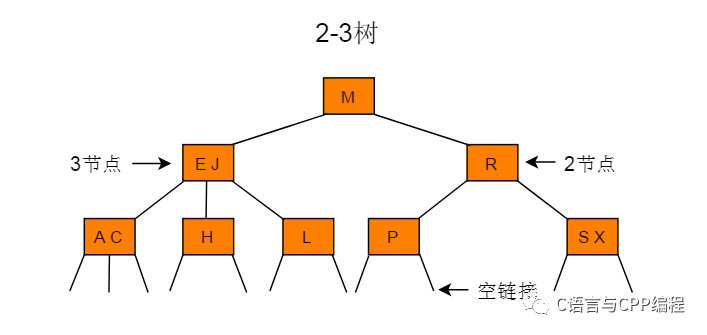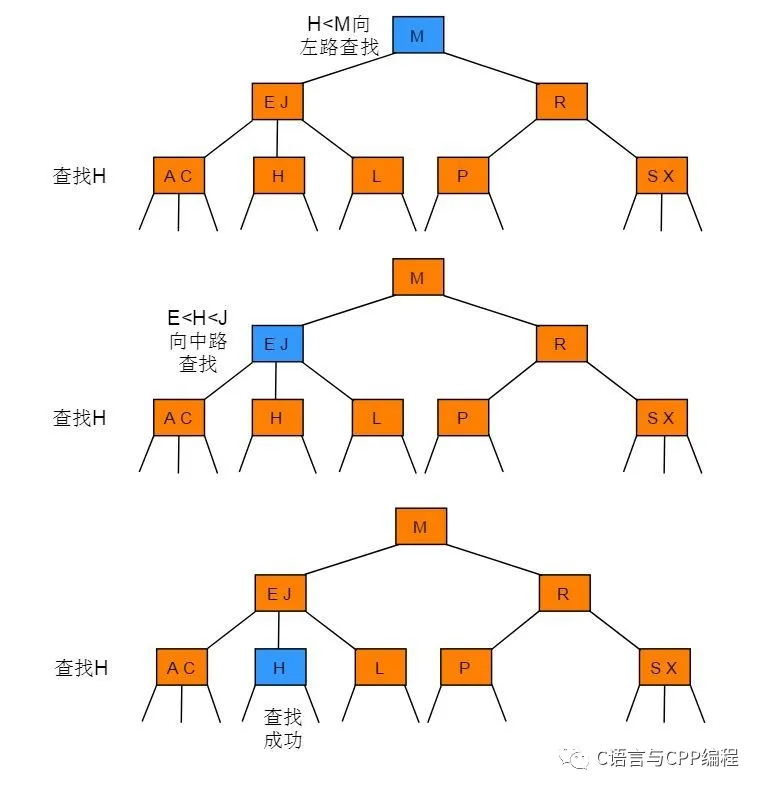2-3 树中查找键为H的节点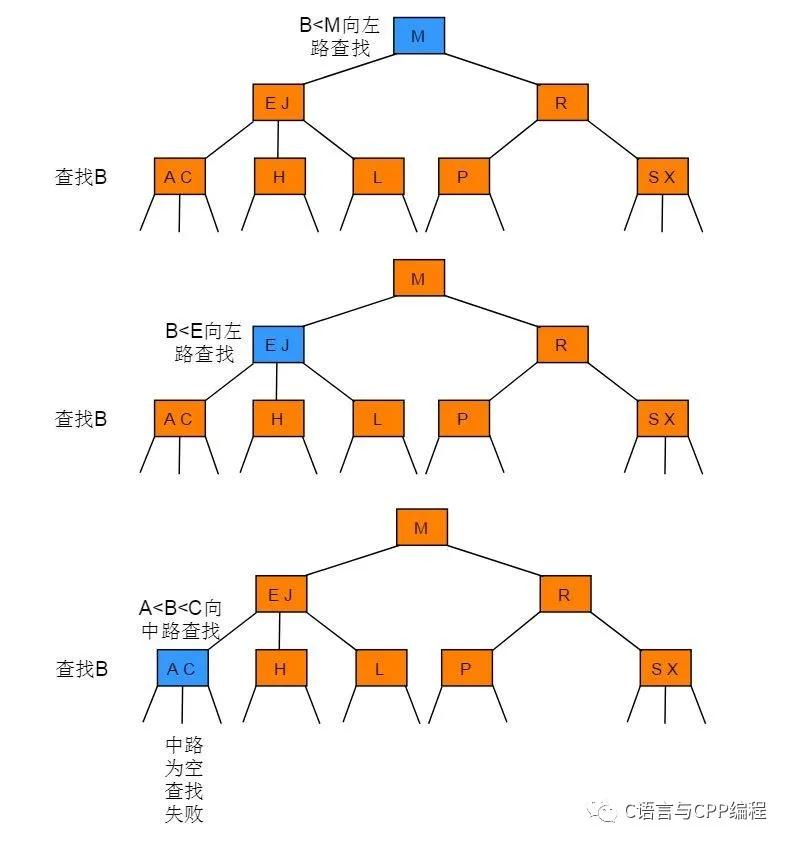2-3 树中查找键为B的节点

two_three *search23(two_three *t, element x){    while(t)    {        if (x < t->data_l)        {            t = t->left_child;        }        else if (x > t->data_l && x < t->data_r)        {            t = t->middle_child;        }        else if (x > t->data_r)        {            t = t->right_child;        }        else        {            return t;        }    }    return NULL;}

## 8 红黑树

2-3查找树能保证在插入元素之后能保持树的平衡状态，最坏情况下即所有的子节点都是2-node，树的高度为lgn，从而保证了最坏情况下的时间复杂度。但是2-3树实现起来比较复杂，于是就有了一种简单实现2-3树的数据结构，即红黑树（Red-Black Tree）。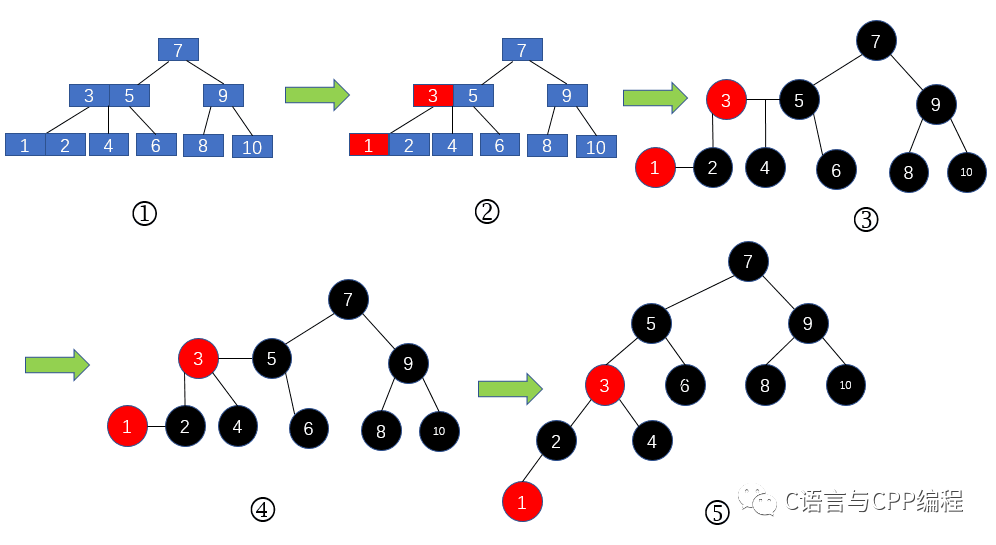2-3树转红黑树

• 红黑树是一种平衡树，他复杂的定义和规则都是为了保证树的平衡性。如果树不保证他的平衡性就是下图：很显然这就变成一个链表了。

• 保证平衡性的最大的目的就是降低树的高度，因为树的查找性能取决于树的高度。所以树的高度越低搜索的效率越高！

• 这也是为什么存在二叉树、搜索二叉树等，各类树的目的。

• 每个节点要么是黑色，要么是红色。

• 根节点是黑色。

• 每个叶子节点（NIL）是黑色。

• 每个红色结点的两个子结点一定都是黑色。

• 任意一结点到每个叶子结点的路径都包含数量相同的黑结点。

#define BLACK 1#define RED 0#include <iostream>using namespace std;class bst{private:	struct Node	{		int value;		bool color;		Node *leftTree, *rightTree, *parent;		Node() : value(0), color(RED), leftTree(NULL), rightTree(NULL), parent(NULL) { }		Node* grandparent()		{			if (parent == NULL)			{				return NULL;			}			return parent->parent;		}		Node* uncle()		{			if (grandparent() == NULL)			{				return NULL;			}			if (parent == grandparent()->rightTree)				return grandparent()->leftTree;			else				return grandparent()->rightTree;		}		Node* sibling()		{			if (parent->leftTree == this)				return parent->rightTree;			else				return parent->leftTree;		}	};	void rotate_right(Node *p)	{		Node *gp = p->grandparent();		Node *fa = p->parent;		Node *y = p->rightTree;		fa->leftTree = y;		if (y != NIL)			y->parent = fa;		p->rightTree = fa;		fa->parent = p;		if (root == fa)			root = p;		p->parent = gp;		if (gp != NULL)		{			if (gp->leftTree == fa)				gp->leftTree = p;			else				gp->rightTree = p;		}	}	void rotate_left(Node *p)	{		if (p->parent == NULL)		{			root = p;			return;		}		Node *gp = p->grandparent();		Node *fa = p->parent;		Node *y = p->leftTree;		fa->rightTree = y;		if (y != NIL)			y->parent = fa;		p->leftTree = fa;		fa->parent = p;		if (root == fa)			root = p;		p->parent = gp;		if (gp != NULL)		{			if (gp->leftTree == fa)				gp->leftTree = p;			else				gp->rightTree = p;		}	}	void inorder(Node *p)	{		if (p == NIL)			return;		if (p->leftTree)			inorder(p->leftTree);		cout << p->value << " ";		if (p->rightTree)			inorder(p->rightTree);	}	string outputColor(bool color)	{		return color ? "BLACK" : "RED";	}	Node* getSmallestChild(Node *p)	{		if (p->leftTree == NIL)			return p;		return getSmallestChild(p->leftTree);	}	bool delete_child(Node *p, int data)	{		if (p->value > data)		{			if (p->leftTree == NIL)			{				return false;			}			return delete_child(p->leftTree, data);		}		else if (p->value < data)		{			if (p->rightTree == NIL)			{				return false;			}			return delete_child(p->rightTree, data);		}		else if (p->value == data)		{			if (p->rightTree == NIL)			{				delete_one_child(p);				return true;			}			Node *smallest = getSmallestChild(p->rightTree);			swap(p->value, smallest->value);			delete_one_child(smallest);			return true;		}		else		{			return false;		}	}	void delete_one_child(Node *p)	{		Node *child = p->leftTree == NIL ? p->rightTree : p->leftTree;		if (p->parent == NULL && p->leftTree == NIL && p->rightTree == NIL)		{			p = NULL;			root = p;			return;		}		if (p->parent == NULL)		{			delete  p;			child->parent = NULL;			root = child;			root->color = BLACK;			return;		}		if (p->parent->leftTree == p)		{			p->parent->leftTree = child;		}		else		{			p->parent->rightTree = child;		}		child->parent = p->parent;		if (p->color == BLACK)		{			if (child->color == RED)			{				child->color = BLACK;			}			else				delete_case(child);		}		delete p;	}	void delete_case(Node *p)	{		if (p->parent == NULL)		{			p->color = BLACK;			return;		}		if (p->sibling()->color == RED)		{			p->parent->color = RED;			p->sibling()->color = BLACK;			if (p == p->parent->leftTree)				rotate_left(p->sibling());			else				rotate_right(p->sibling());		}		if (p->parent->color == BLACK && p->sibling()->color == BLACK			&& p->sibling()->leftTree->color == BLACK && p->sibling()->rightTree->color == BLACK)		{			p->sibling()->color = RED;			delete_case(p->parent);		}		else if (p->parent->color == RED && p->sibling()->color == BLACK			&& p->sibling()->leftTree->color == BLACK && p->sibling()->rightTree->color == BLACK)		{			p->sibling()->color = RED;			p->parent->color = BLACK;		}		else		{			if (p->sibling()->color == BLACK)			{				if (p == p->parent->leftTree && p->sibling()->leftTree->color == RED					&& p->sibling()->rightTree->color == BLACK)				{					p->sibling()->color = RED;					p->sibling()->leftTree->color = BLACK;					rotate_right(p->sibling()->leftTree);				}				else if (p == p->parent->rightTree && p->sibling()->leftTree->color == BLACK					&& p->sibling()->rightTree->color == RED)				{					p->sibling()->color = RED;					p->sibling()->rightTree->color = BLACK;					rotate_left(p->sibling()->rightTree);				}			}			p->sibling()->color = p->parent->color;			p->parent->color = BLACK;			if (p == p->parent->leftTree)			{				p->sibling()->rightTree->color = BLACK;				rotate_left(p->sibling());			}			else			{				p->sibling()->leftTree->color = BLACK;				rotate_right(p->sibling());			}		}	}	void insert(Node *p, int data)	{		if (p->value >= data)		{			if (p->leftTree != NIL)				insert(p->leftTree, data);			else			{				Node *tmp = new Node();				tmp->value = data;				tmp->leftTree = tmp->rightTree = NIL;				tmp->parent = p;				p->leftTree = tmp;				insert_case(tmp);			}		}		else		{			if (p->rightTree != NIL)				insert(p->rightTree, data);			else			{				Node *tmp = new Node();				tmp->value = data;				tmp->leftTree = tmp->rightTree = NIL;				tmp->parent = p;				p->rightTree = tmp;				insert_case(tmp);			}		}	}	void insert_case(Node *p)	{		if (p->parent == NULL)		{			root = p;			p->color = BLACK;			return;		}		if (p->parent->color == RED)		{			if (p->uncle()->color == RED)			{				p->parent->color = p->uncle()->color = BLACK;				p->grandparent()->color = RED;				insert_case(p->grandparent());			}			else			{				if (p->parent->rightTree == p && p->grandparent()->leftTree == p->parent)				{					rotate_left(p);					rotate_right(p);					p->color = BLACK;					p->leftTree->color = p->rightTree->color = RED;				}				else if (p->parent->leftTree == p && p->grandparent()->rightTree == p->parent)				{					rotate_right(p);					rotate_left(p);					p->color = BLACK;					p->leftTree->color = p->rightTree->color = RED;				}				else if (p->parent->leftTree == p && p->grandparent()->leftTree == p->parent)				{					p->parent->color = BLACK;					p->grandparent()->color = RED;					rotate_right(p->parent);				}				else if (p->parent->rightTree == p && p->grandparent()->rightTree == p->parent)				{					p->parent->color = BLACK;					p->grandparent()->color = RED;					rotate_left(p->parent);				}			}		}	}	void DeleteTree(Node *p)	{		if (!p || p == NIL)		{			return;		}		DeleteTree(p->leftTree);		DeleteTree(p->rightTree);		delete p;	}public:	bst()	{		NIL = new Node();		NIL->color = BLACK;		root = NULL;	}	~bst()	{		if (root)			DeleteTree(root);		delete NIL;	}	void inorder()	{		if (root == NULL)			return;		inorder(root);		cout << endl;	}	void insert(int x)	{		if (root == NULL)		{			root = new Node();			root->color = BLACK;			root->leftTree = root->rightTree = NIL;			root->value = x;		}		else		{			insert(root, x);		}	}	bool delete_value(int data)	{		return delete_child(root, data);	}private:	Node *root, *NIL;};int main(){	cout << "---【红黑树】---" << endl;	// 创建红黑树	bst tree;	// 插入元素	tree.insert(2);	tree.insert(9);	tree.insert(-10);	tree.insert(0);	tree.insert(33);	tree.insert(-19);	// 顺序打印红黑树	cout << "插入元素后的红黑树：" << endl;	tree.inorder();	// 删除元素	tree.delete_value(2);	// 顺序打印红黑树	cout << "删除元素 2 后的红黑树：" << endl;	tree.inorder();	// 析构	tree.~bst();	getchar();	return 0;}

## 9 B树/B+树

﻿

B 树可以看作是对2-3查找树的一种扩展，即他允许每个节点有M-1个子节点。

• 定义任意非叶子结点最多只有M个儿子；且M>2；

• 根结点的儿子数为[2, M]；

• 除根结点以外的非叶子结点的儿子数为[M/2, M]；

• 每个结点存放至少M/2-1（取上整）和至多M-1个关键字；（至少2个关键字）

• 非叶子结点的关键字：K, K, …, K[M-1]；且K[i] < K[i+1]；

• 非叶子结点的指针：P, P, …, P[M]；其中P指向关键字小于K的 子树，P[M]指向关键字大于K[M-1]的子树，其它P[i]指向关键字属于(K[i-1], K[i])的子树；

• 所有叶子结点位于同一层；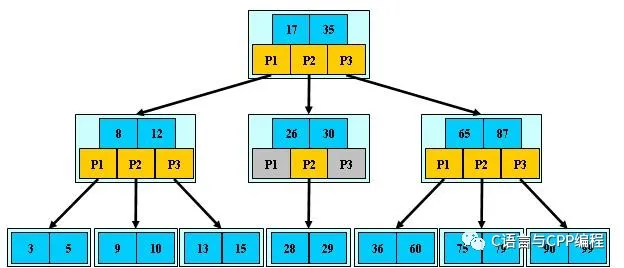﻿

• 关键字集合分布在整颗树中；

• 任何一个关键字出现且只出现在一个结点中；

• 搜索有可能在非叶子结点结束；

• 其搜索性能等价于在关键字全集内做一次二分查找；

• 自动层次控制；

BTNode* BTree_recursive_search(const BTree tree, KeyType key, int* pos){    int i = 0;    while (i < tree->keynum && key > tree->key[i])    {        ++i;    }       // 查找关键字    if (i < tree->keynum && tree->key[i] == key)    {        *pos = i;        return tree;    }       // tree 为叶子节点，找不到 key，查找失败返回    if (tree->isLeaf)    {        return NULL;    }       // 节点内查找失败，但 tree->key[i - 1]< key < tree->key[i]，    // 下一个查找的结点应为 child[i]       // 从磁盘读取第 i 个孩子的数据    disk_read(&tree->child[i]);       // 递归地继续查找于树 tree->child[i]    return BTree_recursive_search(tree->child[i], key, pos);}

B+树

B+树是B树的变体，也是一种多路搜索树：

• 非叶子结点的子树指针与关键字个数相同；

• 非叶子结点的子树指针P[i]，指向关键字值属于[K[i], K[i+1])的子树, B树是开区间

• 为所有叶子结点增加一个链指针;

• 所有关键字都在叶子结点出现；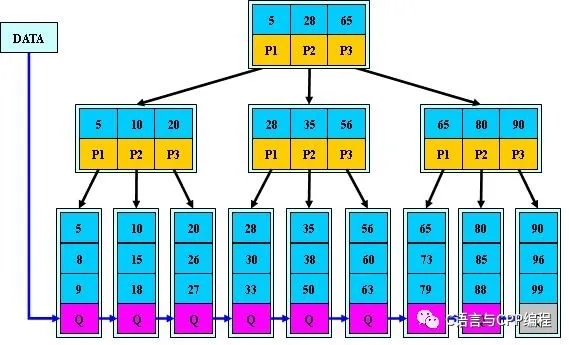1

B+的搜索与B树也基本相同，区别是B+树只有达到叶子结点才命中（B树可以在 非叶子结点命中），其性能也等价于在关键字全集做一次二分查找；

• 所有关键字都出现在叶子结点的链表中（稠密索引），且链表中的关键字恰好是有序的；

• 不可能在非叶子结点命中；

• 非叶子结点相当于是叶子结点的索引（稀疏索引），叶子结点相当于是存储（关键字）数据的数据层；

• 更适合文件索引系统；

## 参考资料

1. https://www.sohu.com/a/296278527_478315

2. https://www.cnblogs.com/exzlc/p/12203744.html

3. 部分配图来源于网络

## 最近原创推荐﻿## 评论